2019-11-04 09:35:19 qq_29440983 阅读数 294
• ###### 机器学习&深度学习系统实战！

购买课程后，可扫码进入学习群，获取唐宇迪老师答疑 数学原理推导与案例实战紧密结合，由机器学习经典算法过度到深度学习的世界，结合深度学习两大主流框架Caffe与Tensorflow，选择经典项目实战人脸检测与验证码识别。原理推导，形象解读，案例实战缺一不可！具体课程内容涉及回归算法原理推导、决策树与随机森林、实战样本不均衡数据解决方案、支持向量机、Xgboost集成算法、神经网络基础、神经网络整体架构、卷积神经网络、深度学习框架--Tensorflow实战、案例实战--验证码识别、案例实战--人脸检测。 专属会员卡优惠链接：http://edu.csdn.net/lecturer/1079

40478 人正在学习 去看看 唐宇迪

## 项目工作环境：

1. python3.5

2. tensorflow-gpu1.9.0

3. beautifulsoup

4. windows10

5. ....

## 数据准备：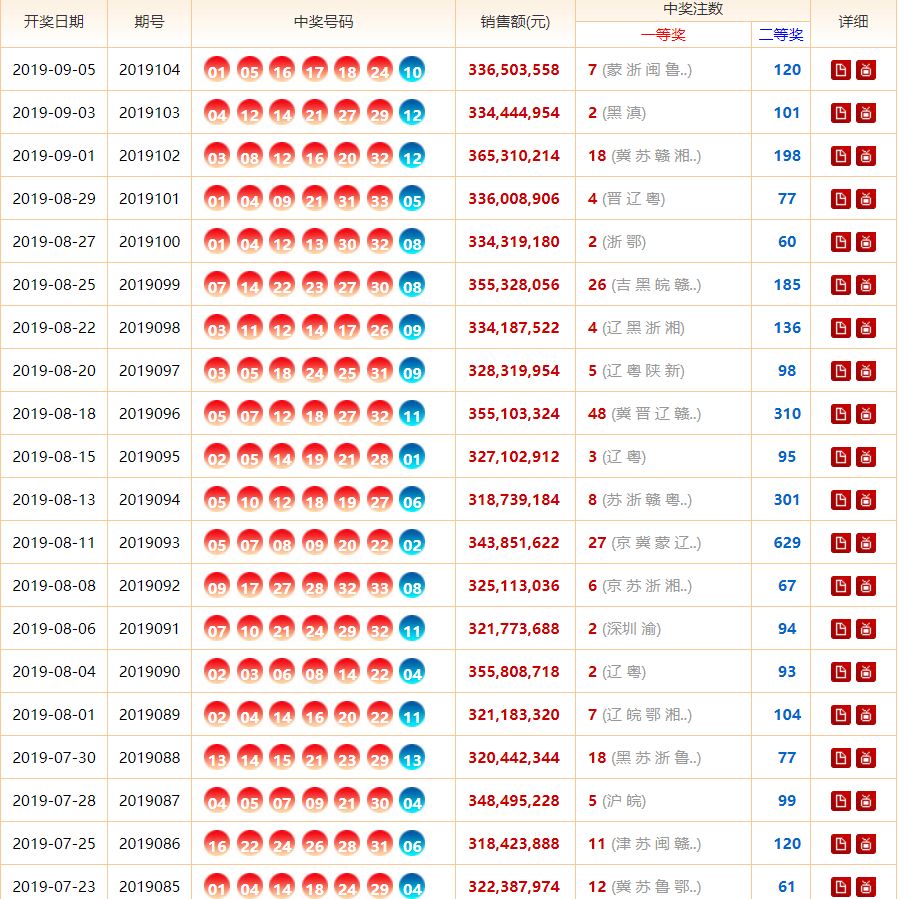1. 对网页源代码进行分析，我们所需要的数据所在代码在如下图，主要需要<td align="center">和<em>标签中的内容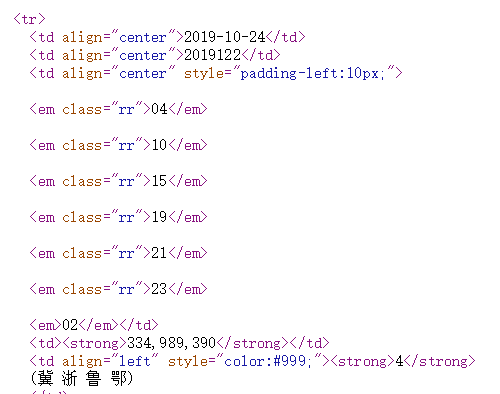2. 因为我们需要爬取出该网站尽可能多的数据，所以还需要中奖结果的总页数，如下图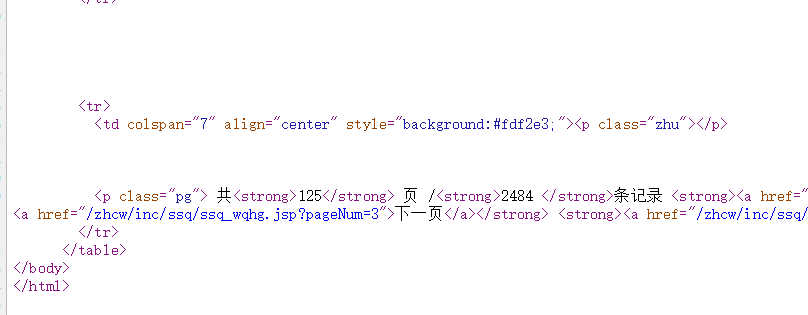3. 使用Python编写爬虫代码，我们主要使用了beautifulsoup来完成数据爬取，主要代码片段如下。
``````#获取该网页的源代码
def getPage(url):
try:
user_agent = 'Mozilla/5.0 (Windows NT 6.2; WOW64) AppleWebKit/537.36 (KHTML, like Gecko) Chrome/27.0.1453.94 Safari/537.36'
response = urllib.request.urlopen(request)
return pageCode
except Exception as e:
print('Get Page Fail')
print("An error has occurred:"+e)

#将双色球的信息写入txt文件
def writePage(date,dateNum,num):
#日期的列表会比期号长1，因为最后加入了一组页数统计，所以不能以日期列表长度来写数据
with open('./DCnumber.txt','a+') as f:
for x in range(0,len(num)):
f.write('('+date[x]+';'+dateNum[x]+';'+num[x]+')'+'\n')
#保存格式为每行('2019-09-24';'2019112';'03,12,14,17,23,27,01')

#获取总页数
def getPageNum(url):
try:
pageCode = getPage(url)
soup = BeautifulSoup(pageCode,'lxml')
td_code = soup.find('td',colspan='7')       #获取表格中包含页数的列
result = td_code.get_text().split(' ')      #将该列转换成列表，我们
#result = ['\n', '共125', '页', '/2484', '条记录', '首页', '上一页', '下一页', '末页', '当前第', '2', '页']
#用正则表达式从reslut中提取出数字
list_num = re.findall("[0-9]{1}",result)
#将提取出来的数字转换成十进制的数值
page_num = int(list_num+list_num+list_num)
return page_num
except Exception as e:
print('Get Page Number Fail')
print("An error has occurred:" + e)

#获取单页中的双色球中奖信息
def getDC(url):
#循环读取每一页的信息
for num in range(1,getPageNum(url)+1):
print('begin get page:'+str(num))
href = 'http://kaijiang.zhcw.com/zhcw/html/ssq/list_'+str(num)+'.html'
page = BeautifulSoup(getPage(href),'lxml')
em_list = page.find_all('em')   #获取该页面中的em内容,即中奖编号所在
td_list = page.find_all('td',{'align':'center'})    #获取该页面中的开奖日期，期号等信息

i = 1   #计数器，每七个号码为一组
DCnum = ''      #存放一期中奖号码
DCnum_list = []     #存放该页每期的中奖号码
for em in em_list:
emnum = em.get_text()
if i == 7:
DCnum = DCnum + emnum
DCnum_list.append(DCnum)
DCnum = ''          #重置一期的号码
i = 1               #重置计数器
else:
DCnum = DCnum + emnum + ','
i += 1

DCdate = []         #存放开奖日期
DCdateN = []        #存放期号
t = 1              #计数器，每5个为一组，我们只需要每组的前两个td内容
for td in td_list:
td_text = td.get_text()
if t == 1:
DCdate.append(td_text)
t += 1
elif t == 2:
DCdateN.append(td_text)
t += 1
elif t == 5:
t = 1
else:
t += 1
writePage(DCdate,DCdateN,DCnum_list)``````

2020-03-07 09:38:54 weixin_40359716 阅读数 35
• ###### 机器学习&深度学习系统实战！

购买课程后，可扫码进入学习群，获取唐宇迪老师答疑 数学原理推导与案例实战紧密结合，由机器学习经典算法过度到深度学习的世界，结合深度学习两大主流框架Caffe与Tensorflow，选择经典项目实战人脸检测与验证码识别。原理推导，形象解读，案例实战缺一不可！具体课程内容涉及回归算法原理推导、决策树与随机森林、实战样本不均衡数据解决方案、支持向量机、Xgboost集成算法、神经网络基础、神经网络整体架构、卷积神经网络、深度学习框架--Tensorflow实战、案例实战--验证码识别、案例实战--人脸检测。 专属会员卡优惠链接：http://edu.csdn.net/lecturer/1079

40478 人正在学习 去看看 唐宇迪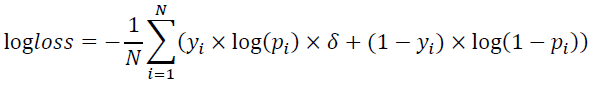yi 表示测试集中 第i个样本的真实标签，
pi 表示第 i个样本的预估转化率，
δ 为惩罚系数。
AB榜的划分方式和比例：
【1】评分采用AB榜形式。排行榜显示A榜成绩，竞赛结束后2小时切换成B榜单。B榜成绩以选定的两次提交或者默认的最后两次提交的最高分为准，最终比赛成绩以B榜单为准。

【2】此题目的AB榜数据采用 同时段数据 是随机划分，A榜为随机抽样测试集50%数据，B榜为其余50%的数据。

https://pan.baidu.com/s/1cPX5jPCuOLDWkEGtCg6OcQ

• 赛题分析
• 数据去操
• 特征选择、构造：挑选有用的特征，生成一些间接的特征（https://zhuanlan.zhihu.com/p/32749489）
• 模型选择
• 结果评估
四、特征处理
我们总共构建了75个指标，其中包括52个基本指标，23个重要指标的变换，平方，对数

``````# 此处只是列举了特征选取的一部分
for idx, data in enumerate([train_last, train_all]):
customer_all = pd.DataFrame(data[['customer_id']]).drop_duplicates(['customer_id']).dropna()
data = data.sort_values(by=['customer_id', 'order_pay_time'])
data['count'] = 1
# 一、购买次数
tmp = data.groupby(['customer_id'])['count'].agg({'customer_counts': 'count'}).reset_index()
customer_all = customer_all.merge(tmp, on=['customer_id'], how='left')
# 二、 省份 , last() 由迭代式获取其中的值， reset_index() 重置索引
tmp = data.groupby(['customer_id'])['customer_province'].last().reset_index()
customer_all = customer_all.merge(tmp, on=['customer_id'], how='left')
# 三、城市
tmp = data.groupby(['customer_id'])['customer_city'].last().reset_index()
customer_all = customer_all.merge(tmp, on=['customer_id'], how='left')
# 四、long_time ： 在train 训练集中的 last - first 的时长, order_pay_date_last : 统计这个用户的订单最后一次购买时间
last_time = data.groupby(['customer_id'], as_index=False)['order_pay_time'].agg(
{'order_pay_date_last': 'max', 'order_pay_date_first': 'min'}).reset_index()
tmp['long_time'] = pd.to_datetime(last_time['order_pay_date_last']) - pd.to_datetime(last_time['order_pay_date_first'])
tmp['long_time'] = tmp['long_time'].dt.days + 1
del tmp['customer_city']
customer_all = customer_all.merge(tmp, on=['customer_id'], how='left')
``````

``````    # 解决倾斜特征
numeric_dtypes = ['int16', 'int32', 'int64', 'float16', 'float32', 'float64']
numeric = []
for i in last.columns:
if last[i].dtype in numeric_dtypes:
numeric.append(i)
``````

``````    import seaborn as sns
import matplotlib.pyplot as plt
sns.set_style('white')
f, ax = plt.subplots(figsize=(8, 7))
ax.set_xscale('log')
ax = sns.boxplot(data=last[numeric], orient='h', palette='Set1')
ax.xaxis.grid(False)
ax.set(ylabel='Feature names')
ax.set(xlabel='Numeric values')
ax.set(title='Numeric Distribution of Features')
sns.despine(trim=True, left=True)
``````

``````  # 寻找偏弱的特征
from scipy.stats import skew, norm

skew_features = last[numeric].apply(lambda x: skew(x)).sort_values(ascending=False)
high_skew = skew_features[skew_features > 0.5]
skew_index = high_skew.index
skewness = pd.DataFrame({'Skew': high_skew})

# 用scipy函数boxcox1p来计算Box-Cox转换。我们的目标是找到一个简单的转换方式使数据规范化。
from scipy.special import boxcox1p
from scipy.stats import boxcox_normmax

for i in skew_index:
last[i] = boxcox1p(last[i], boxcox_normmax(last[i] + 1))

# 处理所有的 skewed values
sns.set_style('white')
f, ax = plt.subplots(figsize=(8, 7))
ax.set_xscale('log')
ax = sns.boxplot(data=last[skew_index], orient='h', palette='Set1')
ax.xaxis.grid(False)
ax.set(ylabel='Feature names')
ax.set(xlabel='Numeric values')
``````

``````    def logs(res, ls):
m = res.shape
for l in ls:
res = res.assign(newcol=pd.Series(np.log(1.01 + res[l])).values)
res.columns.values[m] = l + '_log'
m += 1
return res

def squares(res, ls):
m = res.shape
for l in ls:
res = res.assign(newcol=pd.Series(res[l] * res[l]).values)
res.columns.values[m] = l + '_sq'
m += 1
return res
``````

``````# 由 loss 值计算score
def re_loglossv(labels,preds):
deta = 3.45
y_true = labels   # you can try this eval metric for fun
y_pred = preds
p = np.clip(y_pred, 1e-10, 1-1e-10)
loss = -1/len(y_true) * np.sum(y_true * np.log(p) * deta + (1 - y_true) * np.log(1-p))
return 're_logloss',loss,False
``````

XGB模型参数设置：

``````    import xgboost as xgb
# xgb 模型
from sklearn.model_selection import KFold, RepeatedKFold, StratifiedKFold
folds = StratifiedKFold(n_splits=5, shuffle=True, random_state=2019)
# xgb模型参数设置
xgb_params = {"booster": 'gbtree',
'eta': 0.005,
'max_depth': 5,
'subsample': 0.7,
'colsample_bytree': 0.8,
'objective': 'binary:logistic',
'eval_metric': 'logloss',
'silent': True,
'scale_pos_weight': 2.5   # 处理正负样本不均衡
}

# -----------------------------------------------------------------------------------------------------------

oof_xgb = np.zeros(len(X_train))
predictions_xgb = np.zeros(len(X_valid))
watchlist = [(xgb.DMatrix(X_train.as_matrix(), y_train.as_matrix()), 'train'),
(xgb.DMatrix(X_valid.as_matrix(), y_valid.as_matrix()), 'valid_data')]
clf = xgb.train(dtrain=xgb.DMatrix(np.array(X_train), np.array(y_train)), num_boost_round=500, evals=watchlist,
early_stopping_rounds=200,
verbose_eval=100, params=xgb_params, feval=myFeval)
oof_xgb = clf.predict(xgb.DMatrix(X_valid.as_matrix()), ntree_limit=clf.best_ntree_limit)
pred_xgb = clf.predict(xgb.DMatrix(X_all.as_matrix()), ntree_limit=clf.best_ntree_limit)
res = all_data[['customer_id']]
res['result'] = pred_xgb
# 保存 xgb模型
# clf.save_model('./xgb.model_true_false')
# bst2 = xgb.Booster(model_file='xgb.model1')
``````

``````  # 五折交叉验证
for fold_, (trn_idx, val_idx) in enumerate(folds.split(train_x, train_y)):
print("fold n°{}".format(fold_ + 1))
trn_data = xgb.DMatrix(train_x[trn_idx], train_y[trn_idx])
val_data = xgb.DMatrix(train_x[val_idx], train_y[val_idx])
watchlist = [( trn_data, 'train'), (val_data, 'valid_data')]
clf = xgb.train(dtrain=trn_data, num_boost_round=300, evals=watchlist, early_stopping_rounds=200,
verbose_eval=100, params=xgb_params, feval=myFeval)
oof_xgb[val_idx] = clf.predict(xgb.DMatrix(train_x[val_idx]), ntree_limit=clf.best_ntree_limit)
pred_xgb += clf.predict(xgb.DMatrix(X_all.as_matrix()), ntree_limit=clf.best_ntree_limit) / folds.n_splits
``````

2020-04-01 13:09:20 weixin_43341045 阅读数 56
• ###### 机器学习&深度学习系统实战！

购买课程后，可扫码进入学习群，获取唐宇迪老师答疑 数学原理推导与案例实战紧密结合，由机器学习经典算法过度到深度学习的世界，结合深度学习两大主流框架Caffe与Tensorflow，选择经典项目实战人脸检测与验证码识别。原理推导，形象解读，案例实战缺一不可！具体课程内容涉及回归算法原理推导、决策树与随机森林、实战样本不均衡数据解决方案、支持向量机、Xgboost集成算法、神经网络基础、神经网络整体架构、卷积神经网络、深度学习框架--Tensorflow实战、案例实战--验证码识别、案例实战--人脸检测。 专属会员卡优惠链接：http://edu.csdn.net/lecturer/1079

40478 人正在学习 去看看 唐宇迪

# 前言

去年大二上学期的时候，突然想尝试一下神经网络与深度学习的学习。毕竟博主是想以后做计算机视觉的，于是找我的导师闻了一下相关的学习方法，于是导师给了我一个预测判断牧场动物行为的项目。说实话，一开始心里还是挺没谱的，毕竟我还啥都不会啊ToT。不过人都不是从0到1的质变提升吗？所以我自己就买了一堆的书。在这里我是比较推荐

1. ‘中国水利水电出版社’刘瑜先生的《Python编程》
2. ‘中国水利水电出版社’蒋子阳先生的《TensorFlow深度学习算法原理与编程实战》
3. ‘人民邮电出版社’斋藤康毅先生的《深度学习入门》
4. ‘人民邮电出版社’塔里克·拉希德先生的《Python神经网络编程》
5. ‘人民邮电出版社’IAN GOODFELLOW,YOSHUA BENGIO,AARON COURVILLE三位联合著写的《DEEP LEARNING》

关于视频课程的话，我推荐去B站的莫烦Python，吴恩达先生的视频，以及3Blue1Brown等等。

# 壹.关于各种库的安装

其实在我很多博客里面都有说过，如果还是不会安装库的同学请自行BaiDu或者阅读我之前的python博客。

``````import numpy as np#numpy做矩阵运算十分方便
import math#math函数用来设置一些激活函数
import random#需要随机数来做初始权重
import string
import matplotlib as mpl#如果你需要作图就用上
import matplotlib.pyplot as plt
import sys#需要保存最后的合理权重
import xlrd#因为我的数据集是从excel里面读出来的
from sklearn import preprocessing#需要对数据集做高斯分布或归一化的可以用这个``````

### 读取数据

我这里是用的来自牧场方面给的Excel里面的各项数据集，如果是用MNIST或者其他来源的数据集的朋友可以跳过该步骤。差不多是一个三维的数据，我每次从两种行为里面取500组数据放入数组，并且给他们贴上0或1的标签【【【954】，【4652】，【-456】】【1】】差不多就是这种，前面是三维列表，后面是贴上的标签。

``````def read_excel():
# 打开Excel
workbook = xlrd.open_workbook('all.xls')
# 进入sheet
eating = workbook.sheet_by_index(0)
sleeping=workbook.sheet_by_index(1)
# 获取行数和列叔
Srows_num=sleeping.nrows
Scols_num=sleeping.ncols
ListSleep=[]

Erows_num = eating.nrows
Ecols_num = eating.ncols
ListEat=[]
ListEatT=[]
ListSleepT=[]
ListT=[]
i = 1
while i <= 500:
if Erows_num > 10 or Srows_num>10:
# 生成随机数
random_numE = random.randint(1, Erows_num - 1)
E_X = eating.row(random_numE).value
E_Y = eating.row(random_numE).value
E_Z = eating.row(random_numE).value
ListEat.append([[int(E_X),int(E_Y),int(E_Z)],])
random_numS = random.randint(1, Srows_num - 1)
S_X = sleeping.row(random_numS).value
S_Y = sleeping.row(random_numS).value
S_Z = sleeping.row(random_numS).value
ListSleep.append([[int(S_X),int(S_Y),int(S_Z)],])
i = i + 1
else:
print("数据不足，请添加")
break
List=ListEat+ListSleep

print(List)

# 贰.激活函数

这里是最重要的一步，一个好的适用的激活函数决定你这个网络的质量。

### 1、sigmoid函数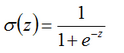sigmoid函数曲线如下: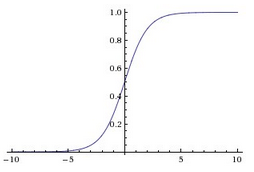sigmoid激活函数，符合实际，当输入值很小时，输出接近于0；当输入值很大时，输出值接近于1。但sigmoid激活函数有较  大的缺点，是主要有两点：

（1）容易引起梯度消失。当输入值很小或很大时，梯度趋向于0，相当于函数曲线左右两端函数导数趋向于0。

（2）非零中心化，会影响梯度下降的动态性。

``````def sigmoid(x):
'''
定义sigmoid函数
'''
return 1.0/(1.0+np.exp(-x))
def derived_sigmoid(x):
'''
定义sigmoid导函数
'''
return sigmoid(x)*(1-sigmoid(x))``````

### 2、tanh函数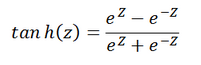tanh函数曲线如下：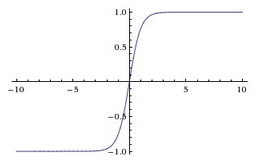与sigmoid相比，输出至的范围变成了0中心化[-1, 1]。但梯度消失现象依然存在。

``````def tanh(x):
'''
定义tanh函数
'''
return (np.exp(x)-np.exp(-x))/(np.exp(x)+np.exp(-x))
def derived_tanh(x):
'''
定义tanh导函数
'''
return 1-tanh(x)*tanh(x)``````

### 3、Relu函数

Relu修正线性单元是有许多优点，是目前神经网络中使用最多的激活函数。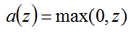函数曲线如下：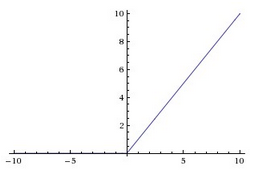（2）前向计算量小，只需要计算max(0, x)，不像sigmoid中有指数计算；

（3）反向传播计算快，导数计算简单，无需指数、出发计算；

（4）有些神经元的值为0，使网络具有saprse性质，可减小过拟合。

``````def relu(x):
'''relu函数'''
return np.where(x<0,0,x)

def derivedrelu(x):
'''relu的导函数'''
return np.where(x<0,0,1)``````

### 4、Leak Relu函数

Leak Relu是对Relu缺点的改进，当输入值小于0时，输出值为αx，其中α是一个很小的常数。这样在反向传播中就不容易出现“die”的情况。

``````def leakyrelu(x,a=0.01):
'''
定义leakyrelu函数
leakyrelu激活函数是relu的衍变版本，主要就是为了解决relu输出为0的问题
'''
return np.where(x<0,a*x,x)

def derived_leakyrelu(x,a=0.01):
'''
定义leakyrelu导函数
'''
return np.where(x<0,a,1)``````
``````

```
```

4、elu函数

`````` # 定义elu函数elu和relu的区别在负区间，relu输出为0，而elu输出会逐渐接近-α，更具鲁棒性。
def elu(x,a=0.01):
#elu激活函数另一优点是它将输出值的均值控制为0（这一点确实和BN很像，BN将分布控制到均值为0，标准差为1）
return np.where(x<0,a*(np.exp(x)-1),x)

def derived_elu(x,a=0.01):
'''
定义elu导函数
'''
return np.where(x<0,a*np.exp(x),1)``````

除此之外还有很多派生的激活函数，请各位自己发掘了。

# 叁.数据的归一化处理

输入输出数据的预处理：尺度变换。尺度变化也称为归一化或者标准化，是指变换处理将网络的输入、输出数据限制在[0,1]或者[-1,1]等区间内。进行变换的原因有三点：

1）.网络的各个输入数据常常具有不同的物理意义和不同的量纲。尺度变换使所有分量都在一个区间内变化，从而使网络训练一开始就给各输入分量以同等重要的地位；

2）.BP神经网络神经元均采用sigmoid函数，变换后可防止因净输入的绝对值过大而使神经元输出饱和，继而使权值调整进入误差曲面的平坦区；

3）.sigmoid函数输出在区间[0,1]或者是tanh函数[-1,1]内，如果不对期望输出数据进行变换处理，势必使数值大的分量绝对误差大，数值小的分量绝对误差小，总之数据最好要归一化。

### 2、（0,1)标准化：

这是最简单也是最容易想到的方法，通过遍历feature vector里的每一个数据，将Max和Min的记录下来，并通过Max-Min作为基数（即Min=0，Max=1）进行数据的归一化处理：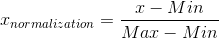Python实现：

``````def MaxMin(x,Max,Min):
x = (x - Min) / (Max - Min);
return x``````

### 2、Z-score标准化：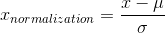Python实现：

``````def Z_Score(x,mu,sigma):
x = (x - mu) / sigma;
return x``````

### 3、均值归一化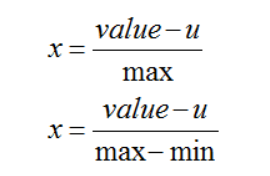``````def average():
# 均值
average = float(sum(data))/len(data)

# 均值归一化方法
data2_1 = [(x - average )/max(data) for x in data]
data2_2 = [(x - average )/(max(data) - min(data)) for x in data]``````

# 肆.误差函数/损失函数

### 一、均方误差Mean Squared Erroryk​是NN的输出
tk​是标签，只有正确解的标签为1，其他均为0.   k是数据维数

``````def get_standard_deviation(records):
"""
标准差 == 均方差 反映一个数据集的离散程度
"""
variance = get_variance(records)
return math.sqrt(variance)``````

二、平均值average

``````def get_average(records):
"""
平均值
"""
return sum(records) / len(records)``````

### 三、交叉熵误差Cross Entropy Error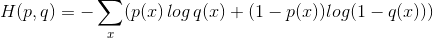yk​是NN的输出tk​标签，只有正确解的标签为1，其他均为0，k是数据维数

``````def cross_entropy(a, y):
return np.sum(np.nan_to_num(-y*np.log(a)-(1-y)*np.log(1-a)))

# tensorflow version
loss = tf.reduce_mean(-tf.reduce_sum(y_*tf.log(y), reduction_indices=))

# numpy version
loss = np.mean(-np.sum(y_*np.log(y), axis=1))``````

# 伍.构建神经网络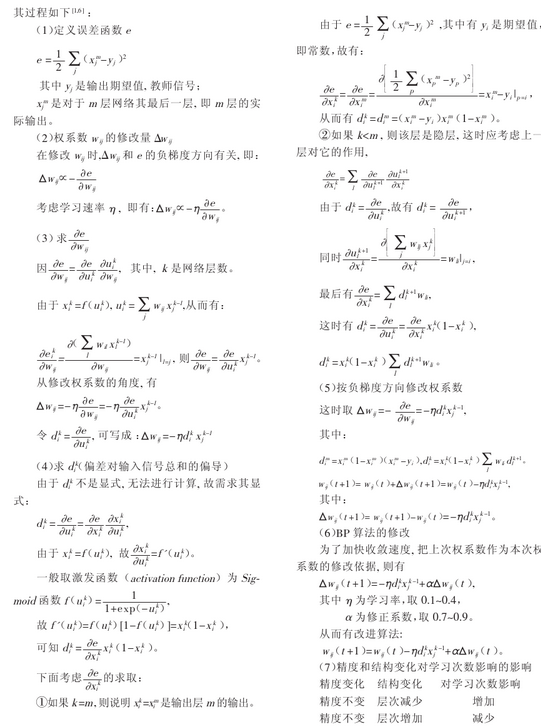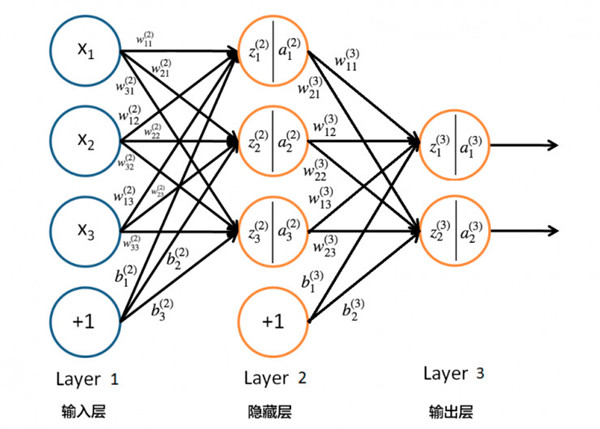这上面的图只是一个示例，我最后的输出只有一个输出点，通过判断接近0或者1来判断行为的，多分类问题建议最后用softmax。这里的初始权值我有必要先说一下，最好设置为2的倍数，特别是要GPU加速的同学。

``````# 构造三层BP网络架构
``````
``````class BPNN:
def __init__(self, num_in, num_hidden, num_out):
# 输入层，隐藏层，输出层的节点数
self.num_in = num_in + 1  # 增加一个偏置结点
self.num_hidden = num_hidden + 1  # 增加一个偏置结点
self.num_out = num_out
# 激活神经网络的所有节点（向量）
self.active_in = [1.0] * self.num_in
self.active_hidden = [1.0] * self.num_hidden
self.active_out = [1.0] * self.num_out
# 创建权重矩阵
self.wight_in = makematrix(self.num_in, self.num_hidden)
self.wight_out = makematrix(self.num_hidden, self.num_out)
# 对权值矩阵赋初值

for i in range(self.num_in):
for j in range(self.num_hidden):
self.wight_in[i][j] = random_number(-2.4, 2.4)
for i in range(self.num_hidden):
for j in range(self.num_out):
self.wight_out[i][j] = random_number(-0.2, 0.2)
# 最后建立动量因子（矩阵）
self.ci = makematrix(self.num_in, self.num_hidden)
self.co = makematrix(self.num_hidden, self.num_out)``````

正向传播，这里我用了sigmoid作为初始数据集的归一化，因为我这里的数据最大和最小差的太多1000和3这种差距，像这种数据之间有太大的差异一定要归一化。tanh作为隐含层的激活函数，把隐含层的数据都统一在[-1,1]之间。最后用sigmoid输出判断这个数据靠近1还是靠近0。

``````   def update(self, inputs):
print(len(inputs))
if len(inputs) != self.num_in - 1:
raise ValueError('输入层节点数有误')
# 数据输入输入层
for i in range(self.num_in - 1):
self.active_in[i] = sigmoid(inputs[i])  #在输入层进行数据处理归一化

self.active_in[i] = inputs[i]  # active_in[]是输入数据的矩阵
# 数据在隐藏层的处理
for i in range(self.num_hidden - 1):
sum = 0.0
for j in range(self.num_in):
sum = sum + self.active_in[i] * self.wight_in[j][i]

self.active_hidden[i] = tanh(sum)  # active_hidden[]是处理完输入数据之后存储，作为输出层的输入数据

# 数据在输出层的处理
for i in range(self.num_out):
sum = 0.0
print(self.wight_out)
for j in range(self.num_hidden):

sum = sum + self.active_hidden[j] * self.wight_out[j][i]
self.active_out[i] = sigmoid(sum)  # 与上同理
return self.active_out[:]``````
```` `
```

反向传播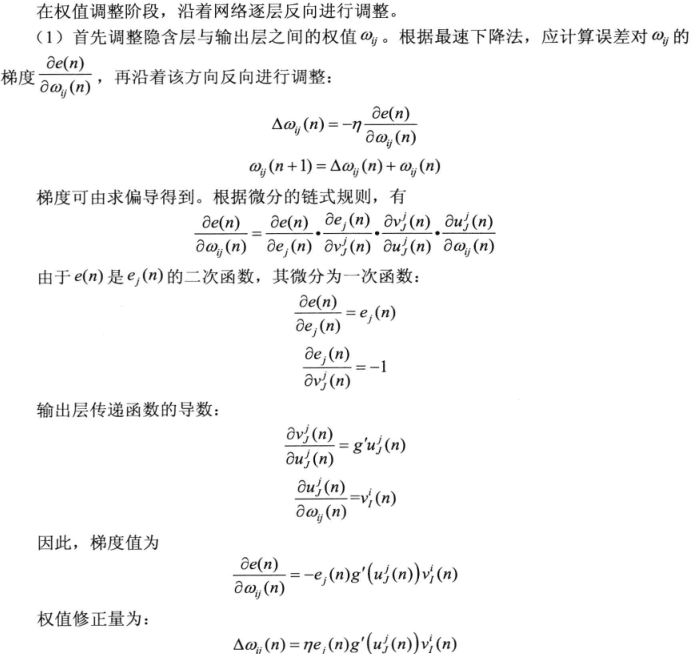``````# 误差反向传播
def errorback(self, targets, lr, m):  # lr是学习率， m是动量因子
if len(targets) != self.num_out:
raise ValueError('与输出层节点数不符！')
# 首先计算输出层的误差
out_deltas = [0.0] * self.num_out
for i in range(self.num_out):
error = targets[i] - self.active_out[i]
out_deltas[i] = derived_sigmoid(self.active_out[i]) * error

# 然后计算隐藏层误差
hidden_deltas = [0.0] * self.num_hidden
for i in range(self.num_hidden):
error = 0.0
for j in range(self.num_out):
error = error + out_deltas[j] * self.wight_out[i][j]
hidden_deltas[i] = derived_tanh(self.active_hidden[i]) * error

# 首先更新输出层权值
for i in range(self.num_hidden):
for j in range(self.num_out):
change = out_deltas[j] * self.active_hidden[i]
self.wight_out[i][j] = self.wight_out[i][j] + lr * change + m * self.co[i][j]
self.co[i][j] = change
# 然后更新输入层权值
for i in range(self.num_in):
for j in range(self.num_hidden):
change = hidden_deltas[j] * self.active_in[i]
self.wight_in[i][j] = self.wight_in[i][j] + lr * change + m * self.ci[i][j]
self.ci[i][j] = change
# 计算总误差
error = 0.0
for i in range(len(targets)):
error = error + 0.5 * (targets[i] - self.active_out[i]) ** 2

return error``````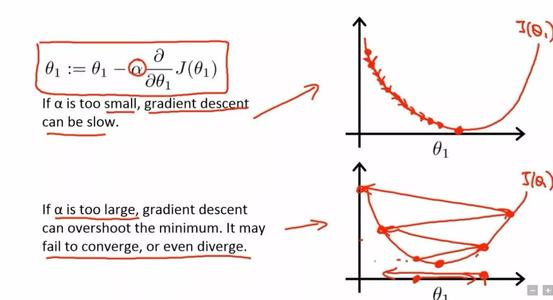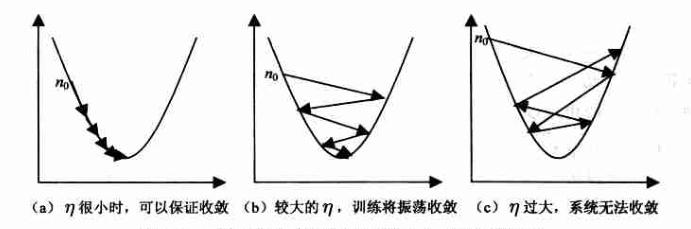BP算法的改进可以使用动量法m

``````  def train(self, pattern, itera=200000, lr=0.1, a=0.5):
for i in range(itera):
error = 0.0
for j in pattern:
input = j
targ = j
self.update(input)
error = error + self.errorback(targ, lr, m)
if i % 200 == 0:
lr*=0.99
print('误差 %-.5f' % error)``````
```` `记录下最后的权重保存在txt文档中
```
``````    def weights(self):
f = open("getwights.txt", "w")
weightin = []
weightout = []
print("输入层权重")
for i in range(self.num_in):
print(self.wight_in[i])
weightin.append(self.wight_in[i])

print("输入矩阵", weightin)
print("输出层权重")
for i in range(self.num_hidden):
print(self.wight_out[i])
weightout.append(self.wight_out[i])

print("输出矩阵", weightout)
f.write(str(weightin))
f.write("\n")
f.write(str(weightout))
f.close()``````

``````def BP():
# 创建神经网络，3个输入节点，3  个隐藏层节点，1个输出层节点
n = BPNN(3, 3, 1)
# 训练神经网络
n.train(List)
# 保存权重值
n.weights()
if __name__ == '__main__':
BP()``````

``````class BPNN:
def __init__(self, num_in, num_hidden, num_out):
# 输入层，隐藏层，输出层的节点数
self.num_in = num_in + 1  # 增加一个偏置结点
self.num_hidden = num_hidden + 1  # 增加一个偏置结点
self.num_out = num_out
# 激活神经网络的所有节点（向量）
self.active_in = [1.0] * self.num_in
self.active_hidden = [1.0] * self.num_hidden
self.active_out = [1.0] * self.num_out
# 创建权重矩阵
self.wight_in = makematrix(self.num_in, self.num_hidden)
self.wight_out = makematrix(self.num_hidden, self.num_out)
# 对权值矩阵赋初值
f = open("getwights.txt", "r")
listone = eval(a)
listtwo = eval(b)
f.close()
for i in range(len(listone)):
for j in range(len(listone[i])):
self.wight_in[i][j] = listone[i][j]
for i in range(len(listtwo)):
for j in range(len(listtwo[i])):
self.wight_out[i][j] = listtwo[i][j]

self.co = makematrix(self.num_hidden, self.num_out)
# 信号正向传播

def update(self, inputs):
if len(inputs) != self.num_in - 1:
raise ValueError('与输入层节点数不符')
# 数据输入输入层
for i in range(self.num_in - 1):
# self.active_in[i] = sigmoid(inputs[i])  #或者先在输入层进行数据处理
self.active_in[i] = inputs[i]  # active_in[]是输入数据的矩阵
# 数据在隐藏层的处理
for i in range(self.num_hidden - 1):
sum = 0.0
for j in range(self.num_in):
sum = sum + self.active_in[i] * self.wight_in[j][i]
self.active_hidden[i] = tanh(sum)  # active_hidden[]是处理完输入数据之后存储，作为输出层的输入数据
# 数据在输出层的处理
for i in range(self.num_out):
sum = 0.0
for j in range(self.num_hidden):
sum = sum + self.active_hidden[j] * self.wight_out[j][i]
self.active_out[i] = sigmoid(sum)  # 与上同理
return self.active_out[:]
# 测试
def test(self, patterns):
distingish=[]
List_update=[]
num=0
sum=0
for i in patterns:
List_update.append(self.update(i))
sum=np.sum(List_update,axis=0)
average=sum/(len(patterns))
#print(average)
for i in patterns:
num += 1
if (num <= testnums and self.update(i) > average):
distingish.append(True)
if (num > testnums and self.update(i) < average):
distingish.append(True)
print(i, '->', self.update(i))
print("正确率", len(distingish) / num)

# 实例
def BP():

# 创建神经网络，3个输入节点，3个隐藏层节点，1个输出层节点
n = BPNN(3, 3, 1)
# 测试神经网络
n.test(ListT)
if __name__ == '__main__':
BP()``````

# 后序

https://blog.csdn.net/weixin_43341045/article/details/104411456

鄙人仅为一名普普通通大二学生，才学浅出，来此各地高人聚集处书写浅见，还望各位前辈高人多多指点海涵。我们诚邀各地有志之士加入我们的代码学习群交流：871352155（无论你会C/C++还是Java，Python还是PHP......有兴趣我们都欢迎你的加入，不过还请各位认真填写加群信息。群内目前多为大学生，打广告的先生女士就请不要步足了。我们希望有远见卓识的前辈能为即将步入社会的初犊提出建议指引方向。）

```
```

2020-03-08 16:03:47 qq_24601199 阅读数 43
• ###### 机器学习&深度学习系统实战！

购买课程后，可扫码进入学习群，获取唐宇迪老师答疑 数学原理推导与案例实战紧密结合，由机器学习经典算法过度到深度学习的世界，结合深度学习两大主流框架Caffe与Tensorflow，选择经典项目实战人脸检测与验证码识别。原理推导，形象解读，案例实战缺一不可！具体课程内容涉及回归算法原理推导、决策树与随机森林、实战样本不均衡数据解决方案、支持向量机、Xgboost集成算法、神经网络基础、神经网络整体架构、卷积神经网络、深度学习框架--Tensorflow实战、案例实战--验证码识别、案例实战--人脸检测。 专属会员卡优惠链接：http://edu.csdn.net/lecturer/1079

40478 人正在学习 去看看 唐宇迪

去年的今天，我就开始有个小小的念头，拥有一个属于自己的博客，其实早在2017年底我就在阿里云购买了属于我自己的第一台服务器，那时的我不知天高地厚，扬言要做一个股票预测系统，并以此为自己的毕业设计，并且还说要把这个系统变成我博客的一个模块（现在想想，那时候真是初出牛犊不怕虎啊，啥都不会，还痴心妄想在短时间内搞定深度学习）。后来的结果是，勉强在老师的帮助下完成了（本科毕设你懂的），能走通流程，换句话就是说能跑得起来，能看得到结果，至于结果的准确性嘛，看看就好，不必在意。

毕业后，准确说工作后三四个月后，开始和老友商量着搞一个系统，先以博客的形式出现，至于系统里面的东西，暂时保密（虽然说八字还没一撇，但总归是策划了，花了心血，以后有时间一定要搞出来，各位看官老爷小小期待一下吧~）。到后来各种各样的事情，我们都没足够的时间，于是乎一直拖（我自己懒还是主要原因），拖到了现在还没正式开始码代码。

一转眼，时间来到了2020年，00后都开始自称老阿姨的年代了，不得不感慨一下，光阴似箭，日月如梭啊。这次下定了决心要弄出来这个博客，于是乎在小破站上找了给150+集的某教育集团的视频，开始了看视频之旅，当然也在各大知识的海洋中大概了解明白了Django的运行流程，自己也跑通了一遍，目前还在加前端模板整合过程中，我会不间断产出搭建过程的博客。

这里我用到的环境如下：

Django 1.11.7

Python 3.6.4

MySQL 5.6.27

PyCharm 2019.3

阿里云服务器（1核 2G）

我的这个项目准备用Django 1.11.7，这里我之所以不用2.2版本，是因为它还刚刚出来，肯定有各种各样的问题，敢说这种肯定的话，是因为我尝试过了，有个目前还没有修复的Bug，引用pymysql模块有问题，网上的解决办法就是降级到2.1.8版本，那我为何不用1.11.x这种LTS版本的呢？至于为什么不用1.8.x版本，你可能会说它也是LTS哇，在我的理念里有这么一句话，用新不用旧，还得用稳定，网上文档多的东西。综上所述，1.11.x版本就是最合适我的啦~（附Django版本发行周期图）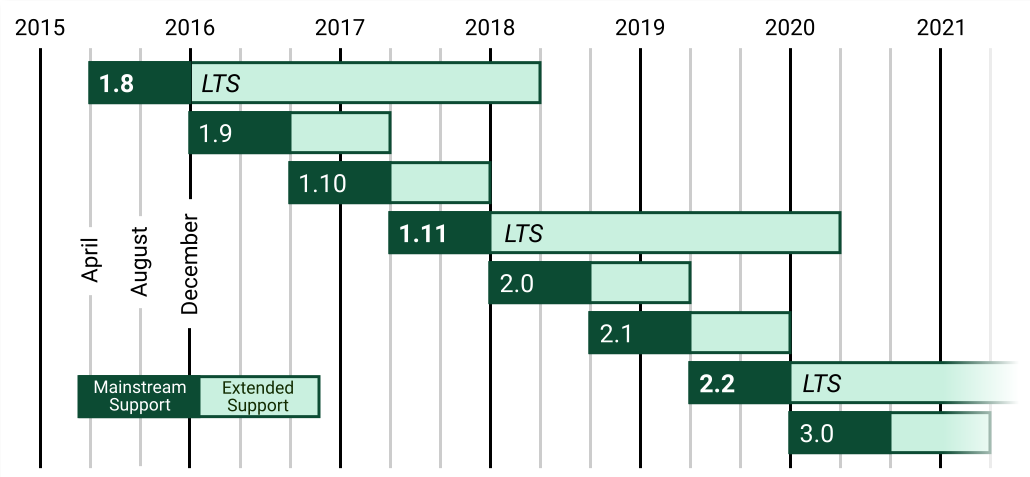说完Django版本的选择，接下来谈谈我为什么选择Python3.6.x吧，其实这也算是有点原因吧，你要知道一个事，Django不同版本对应的可以兼容的Python版本竟然是有要求的，具体对应情况如下，2.7当然是不太建议的了，毕竟从2020年也就是今年开始不再被支持了，其余的版本就看个人喜好咯，至于3.8版本，比较新，选择还请慎重。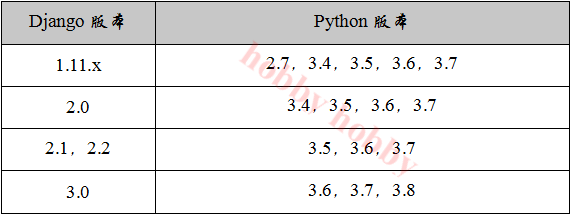2018-11-13 14:13:00 weixin_30787531 阅读数 10
• ###### 机器学习&深度学习系统实战！

购买课程后，可扫码进入学习群，获取唐宇迪老师答疑 数学原理推导与案例实战紧密结合，由机器学习经典算法过度到深度学习的世界，结合深度学习两大主流框架Caffe与Tensorflow，选择经典项目实战人脸检测与验证码识别。原理推导，形象解读，案例实战缺一不可！具体课程内容涉及回归算法原理推导、决策树与随机森林、实战样本不均衡数据解决方案、支持向量机、Xgboost集成算法、神经网络基础、神经网络整体架构、卷积神经网络、深度学习框架--Tensorflow实战、案例实战--验证码识别、案例实战--人脸检测。 专属会员卡优惠链接：http://edu.csdn.net/lecturer/1079

40478 人正在学习 去看看 唐宇迪RNNs维基解释：

https://en.wikipedia.org/wiki/Recurrent_neural_network

LSTM 维基解释：

https://en.wikipedia.org/wiki/Long_short-term_memory

LSTMs能够从时间序列数据中捕捉最重要的特征并进行关联建模。股票价格预测模型是关于对冲基金如何使用此类系统的典型案例，使用了Python编写的PyTorch框架进行训练，设计实验并绘制结果。

• 首先，引入深度学习这个抽象概念。
• 其次，引入RNNs（或更具体地说是LSTMs）以及它们如何进行时间序列分析。
• 接着，让读者熟悉适合深度学习模型的金融数据。
• 接着，举一个实例来说明一支对冲基金如何使用深度学习预测股票价格。
• 最后，就如何使用深度学习来提高对现有或新购对冲基金的表现提供可操作的建议。

Nvidia Tesla维基解释：

https://en.wikipedia.org/wiki/Nvidia_Tesla

• 银行
• 私营企业
• 组织机构
• 提供或出售证券的发行机构的董事，执行官和普通合伙人
• 个人净资产或与该人配偶合资净值超过1,000,000美元的自然人
• 自然人在最近两年每个年度的个人收入超过20万美元，或与该人的配偶每年在该年度的共同收入超过30万美元，并且当年的预期收入也达到相同的水平。
• 信托资产总额超过5,000,000美元
• 所有股权拥有者均为认可投资者的实体1. 数据采集。数据被分成3部分：训练集、验证集和测试集。
2. 使用训练集进行多轮（每一轮包含多个迭代过程）训练DNN模型，并在每轮训练后使用验证集进行验证。
3. 在不断训练和验证后，测试模型（一个带有固定参数的神经网络实例）。LSTMs与一般的循环神经网络结构一样，但是不同的是循环神经元的结构更为复杂。从上图可以看出，在一个LSTM单元内存在大量的计算。

http://colah.github.io/posts/2015-08-Understanding-LSTMs/